Home » NCERT Solutions for Class 12 Accountancy Part II Chapter 5 Accounting Ratios

# NCERT Solutions for Class 12 Accountancy Part II Chapter 5 Accounting Ratios

• State which of the following statements are True or False.
(a) The only purpose of financial reporting is to keep the managers informed about the progress of operations.

(b) Analyses of data provided in the financial statements a is termed as financial analysis.

(c) Long term creditors are concerned about the ability of a firm to discharge its obligations to pay interest and repay the principal amount of term.

(d) A ratio is always expressed as a quotient of one number divided by another.

(e) Ratios help in comparisons of a firm’s results over a number of accounting periods as well as with other business enterprises.

(f) One ratios reflect both quantitative and qualitative aspects.

### DO IT YOURSELF I

Question 1. Current ratio =4.5:1,quick ratio =3:1, Inventory is Rs.36,000. Calculate the current assets and current liabilities.Question 2. Current liabilities of a company are ? 5,60,000 current ratio is 5 : 2 and quick ratio is 2 : 1. Find the value of the stock.Question 3. Current assets of a company are Rs. 5,00,000. Current ratio is 2.5 : 1 and quick ratio is 1 : 1. Calculate the value of current liabilities, liquid assets and stock.(i) The following groups of ratios primarily measure risk
(a) liquidity, activity and profitability
(b) liquidity, activity and common stock
(c) liquidity, activity and debt
(d) activity, debt and profitability
Answer (c) Liquidity, activity and debt

(ii) The————-ratios are primarily measures of return.
(a) liquidity (b) activity
(c) debt (d) profitability

(iii) The…………….of a business firm is measured by its ability to satisfy
its short term obligations as they come due.
(a) activity (b) liquidity
(c) debt (d) profitability

(iv) ……………….ratios are a measure of the speed with which various
accounts are converted into sales or cash.
(a) Activity (b) Liquidity
(c) Debt (d) Profitability

(v) The two basic measure of liquidity are
(a) inventory turnover and current ratio
(b) current ratio and liquid ratio
(c) gross profit margin and operating ratio
(d) current ratio and average collection period
Answer (b) Current ratio and liquid ratio

(vi) The……………is a measure of liquidity which excludes………………….., generally the least liquid asset.
(a) current ratio, accounts debtors
(b) liquid ratio, accounts debtors
(c) current ratio, inventory
(d) liquid ratio, inventory

### DO IT YOURSELF II

Question 1. Calculate the amount of gross profit
Average stock = Rs.80,000
Stock turnover ratio = 6 times
Selling price = 25% above cost
Answer Average stock = ? 80,000
Stock Turnover Ratio = 6 times
Selling Price = 25% above costQuestion 2. Calculate stock Turnover Ratio(i) The………..is useful in evaluating credit and collection policies.
(a) average payment period (b) current ratio
(c) average collection period (d) current asset turnover

(ii) The………measures the activity of a firm’s inventory.
(a) average collection period (b) inventory turnover
(c) liquid ratio (d) current ratio

(iii) The………..ratio may indicate the firm is experiencing stock outs and lost sales.
(a) average payment period (b) inventory turnover
(c) average collection period (d) quick

(iv) ABC Co extends credit terms of 45 days to its customer, its credit collection would be considered poor if its average collection period was
(a) 30 days (b) 36 days
(c) 47 days (d) 57 days

(v) …………… are especially interested in the average payment period, since it provides them with a sense of the bill-paying patterns of the firm.
(a) Customers (b) Stockholders
(c) Lenders and suppliers (d) Borrowers and buyers

(vi) The……………….. ratios provide the information critical to the long-run operation of the firm
(a) liquidity (b) activity
(c) solvency (d) profitability

Question 1. What do you mean by Ratio Analysis?
Answer The ratio analysis is the most powerful tool of financial statement analysis. Ratios simply mean one number expressed in terms of another. A ratio is a statistical yardstick by means of which relationship between two or various figures can be compared or measured. Ratios can be found out by dividing one number by another number. Ratios show how one number is related to another.

Question 2. What are various types of ratios?
Answer Accounting ratios are classified in two ways Categories as follows
(i) Traditional Classification: Traditional ratios are those accounting ratios which are based on the Financial Statement like Trading and Profit and Loss Account and Balance Sheet. On the basis of accounts of financial statements, the Traditional Classification is further divided into the following categories
(a) Income Statement Ratios: like Gross Profit Ratio, etc.
(b) Balance Sheet Ratios: like Current Ratio, Debt Equity Ratio, etc.
(c) Composite Ratios :like Debtors Turnover Ratio, etc.
(ii) Functional Classification This classification of ratios is based on the functional need and the purpose for calculating ratio. The functional ratios are further divided into the following categories
(a) Liquidity Ratio: These ratios are calculated to determine short term solvency.
(b) Solvency Ratio :These ratios are calculated to determine long term solvency.
(c) Activity Ratio :These ratios are calculated for measuring the operational efficiency and efficacy of the operations. These ratios relate to sales or cost of goods sold.
(d) Profitability Ratio: These ratios are calculated to assess the

Question 3. What relationships will be established to study?
(a) Inventory Turnover (b) Debtor Turnover
(c) Payables Turnover (d) Working Capital Turnover
Answer (a) Inventory Turnover Ratio: This ratio is a relationship between the cost of goods sold during a particular period of time and the cost of average inventory during a particular period. It is expressed in number of times. Stock turnover ratio/inventory turnover ratio indicates the number of time the stock has been turned over during the period and evaluates the efficiency with which a firm is able to manage its inventory.
This ratio indicates whether investment in stock is within proper limit or not. The ratio is calculated by dividing the cost of goods sold by the amount of average stock at cost. The formula for calculating inventory turnover ratio is as follows(b)Debtor Turnover Ratio :Debtor turnover ratio or accounts receivable turnover ratio indicates the velocity of debt collection of a firm. In simple words it indicates the number of times average debtors (receivable) are turned over during a year. The formula for calculating Debtors turnover ratio is as follows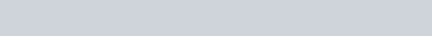(c)Creditors/Payables Turnover Ratio :It compares creditors with the total credit purchases. It signifies the credit period enjoyed by the firm in paying creditors. Accounts payable include both sundry creditors and bills payable. Same as debtor’s turnover ratio, creditor’s turnover ratio can be calculated in two forms, creditors’ turnover ratio and average payment period. The following formula is used to calculate the creditors Turnover Ratio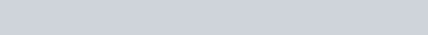(d)Working Capital Turnover Ratio Working capital turnover ratio indicates the velocity of the utilization of net working capital. This ratio represents the number of times the working capital is turned over in a year and is calculated as follows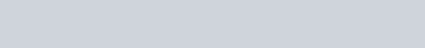Question 4. Why would the inventory turnover ratio be more important when analysing a grocery store than an insurance company?
Answer Nature of business make inventory turnover ratio more important in case of a grocery store as compare to an insurance company. A grocery store is a trading concern involved in trading i.e., buying and selling of goods and in this regards it is obvious to maintain some inventory in stores. On the other hand, insurance company involved in service business and involved in delivering service there is no question of inventory because service is perishable in nature and cannot be stored. That’s why inventory turnover ratio is more important in case of grocery store than an insurance company.

Question 5. The liquidity of a business firm is measured by its ability to satisfy its long term obligations as they become due? Comment.
Answer Yes it is true that the liquidity of a business firm is measured by its ability to pay its long term obligations as they become due. Here the long term obligation means payments of principal amount on the due date and payments of interests on the regular basis. For measuring the long term solvency of any business we calculate the following ratios.

Debt Equity Ratio: Debt equity ratio indicates the relationship between the external equities or outsiders funds and the internal equities or shareholders funds. It is also known as external internal equity ratio. It is determined to ascertain soundness of the long term financial policies of the company. Following formula is used to calculate debt to equity ratio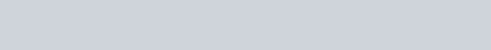Proprietory Ratio/Total Assets to Debt Ratio: Total assets to Debt Ratio or Proprietory Ratio are a variant of the debt equity ratio. It is also known as equity ratio or net worth to total assets ratio. This ratio relates the shareholder’s funds to total assets. Proprietory / Equity ratio indicates the long-term or future solvency position of the business. Formula of Proprietory/Equity Ratio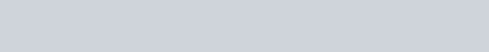Fixed Assets to Proprietor’s Fund Ratio: Fixed assets to proprietor’s fund ratio establish a relationship between fixed assets and shareholders’ funds. The purpose of this ratio is to indicate the percentage of the owner’s funds invested in fixed assets. The formula for calculating this ratio is as follows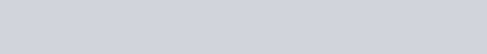Interest Coverage Ratio: This ratio deals only with servicing of return on loan as interest. This ratio depicts the relationship between amount of profit utilise for paying interest and amount of interest payable. A high Interest Coverage Ratio implies that the company can easily meet all its interest obligations out of its profit.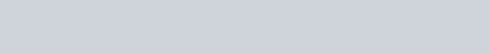Question 6. The average age of inventory is viewed as the average length of time inventory is held by the firm or as the average number of day’s sales in inventory. Explain.
Answer Inventory Turnover Ratio This ratio is a relationship between the cost of goods sold during a particular period of time and the cost of average inventory during a particular period. It is expressed in number of times. Stock turnover ratio/inventory turnover ratio indicates the number of time the stock has been turned over during the period and evaluates the efficiency with which a firm is able to manage its inventory. The formula for calculating inventory turnover ratio is as followsFrom the above formula, it is clear that this ratio reveals the average length of time for which the inventory is held by the firm.

Question 1. Who are the users of financial ratio analysis? Explain the significance of ratio analysis to them.
Answer Financial ratios help their users to take various managerial decisions. In this context there are four categories of users who are interested in financial ratios. These are the management, investors, long term creditors and short term creditors. The significance of ratios to the above mentioned users is as follows
(i) Management :Management calculate ratios for taking various managerial decisions. Management is always interested in future growth of the organisation. In this regard management design various policy measures and draft future plans. Management wish to know how effectively the resources are being utilised conseguently, they are interested in Activity Ratios and Profitability Ratios like Net Profit Ratio, Debtors Turnover Ratio, Fixed Assets Turnover Ratios, etc. ‘
(ii) Equity Investors :The prime concern of investors before investing in shares is to ensure the security of their principle and return on investment. It is a well known fact that the security of the funds is directly related to the profitability and operational efficiency of the business. In this way they are interested in knowing Earnings per Share, Return on Investment and Return on Equity.
(iii) Long Term Creditors: Long term creditors are those creditors who provide funds for more than one year, so they are interested in long term solvency of the firm and in assessing the ability of the firm to pay interest on time. In this way they are interested in calculating Long term Solvency Ratios like, Debt-Equity Ratio, Proprietory Ratio, Total Assets to Debt Ratio, Interest Coverage Ratio, etc.
(iv) Short Term Creditors :Short term creditors are those creditors who provide financial assistance through short term credit (Generally less than one year). That’s why short-term creditors are interested in timely payment of their debts in short run. In this way they are always interested in Liquidity Ratios like, Current Ratio, Quick Ratios etc. These ratios reveal the current financial position of the business. It is always observed that short term obligations are paid through current assest.

Question 2. What are liquidity ratios? Discuss the importance of current and liquid ratio.
Answer Liquidity ratios are calculated to determine the short-term solvency of the business. Analysis of current position of liquid funds determines* the ability of the business to pay the amount due as per commitment to stakeholders. Included in this category are current ratio, Quick ratio and Cash Fund Ratios.

Current Ratio/Working Capital Ratio: This ratio establish relationship between current assets and current liabilities. The standard for this ratio is 2 : 1. It means a ratio 2 : 1 is considered favourable. It is calculated by dividing the total of the current assets by total of the current liabilities. The formula for the current ratio is as follows
Current Ratio = Current Assets/Current Liabilities Or
Current Assets : Current Liabilities
Importance of Current Ratio Current Ratio Provides a measure of degree to which current assets cover current liabilities. The excess of current assets over current liabilities provides a measure of safety margin available against uncertainty in realisation of current assets and flow of funds. However, it must be interpreted carefully because window-dressing is possible by manipulating the components of current assets and current liabilities, e.g., it can be manipulated by making payment to creditors. A very high current ratio is not a good sign as it reflects under utilisation or improper utilisation of resources.
Liquid/Acid Test/Quick Ratio This ratio establishes relationship between Quick assets and Current liabilities. Quick assets are those assets which can get converted into cash easily in case of emergency. Out of current assets it is believed that stock, and prepaid expenses are not possible to convert in cash quickly. The standard for this ratio is 1:1. It means if quick assets are just equal to the current liabilities they will be considered favourable with the view point of company’s credibility. The formula for the quick ratio is as followsImportance of Current Ratio: Current Ratio Provides a measure of degree to which current assets cover current liabilities. The excess of current assets over current liabilities provides a measure of safety margin available against uncertainty in realisation of current assets and flow of funds. However, it must be interpreted carefully because window-dressing is possible by manipulating the components of current assets and current liabilities, e.g., it can be manipulated by making payment to creditors. A very high current ratio is not a good sign as it reflects under utilisation or improper utilisation of resources.
Liquid/Acid Test/Quick Ratio:This ratio establishes relationship between Quick assets and Current liabilities. Quick assets are those assets which can get converted into cash easily in case of emergency. Out of current assets it is believed that stock, and prepaid expenses are not possible to convert in cash quickly. The standard for this ratio is 1:1. It means if quick assets are just equal to the current liabilities they will be considered favourable with the view point of company’s credibility. The formula for the quick ratio is as followsImportance of Quick Ratio :It helps in determining whether a firm has sufficient funds if it has to pay all its current liabilities immediately. Because of exclusion of non-liquid current assets, it is considered better than current ratio as a measure of liquidity position of the business. Standard for liquid ratio is 1:1. Sometimes quick ratio is calculated on the basis of quick liability instead of current liabilities. Quick liabilities are calculated by ignoring bank overdraft, if any. It means to get the figure of quick liabilities from current liabilities; bank overdraft is deducted from current liabilities.

Question 3. How would you study the solvency position of the firm?
Answer The solvency position of any firm is determined and measured with the help of solvency ratios. In this way we can say that the ratios which throw light on the debt servicing ability of the businesses in the long run are known as solvency ratios. Solvency of a concern can be measured in two ways first to check the security of Debt and second is to check the security of return on Debt. For calculating the security of debt we calculate Debt-Equity Ratio, Proprietory Ratio, Fixed Assets – Proprietory Fund Ratio, etc. And for calculating Security of Return on Debt we calculate Interest Coverage Ratio. A brief description of the above mentioned ratios is as follows

Debt Equity Ratio :Debt Equity Ratio indicates the relationship between the external equities or outsiders funds and the internal equities or shareholders funds. It is also known as external internal equity ratio. It is determined to ascertain soundness of the long term financial policies of the company.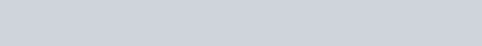Proprietory Ratio/ Total Assets to Debt Ratio: Total assets to Debt Ratio or Proprietory Ratio are a variant of the debt equity ratio. It is also known as equity ratio or net worth to total assets ratio. This ratio relates the shareholder’s funds to total assets. Proprietory/Equity Ratio indicates the long-term or future solvency position of the business. Formula of Proprietary/Equity Ratio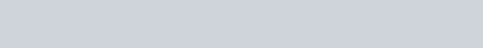Shareholder’s funds include equity share capital plus all reserves and surpluses items. Total assets include all assets, including Goodwill. Some authors exclude goodwill from total assets. In that case the total shareholder’s funds are to be divided by total tangible assets. The total liabilities may also be used as the denominator in the above formula.

Fixed Assets to Proprietor’s Fund Ratio: Fixed Assets to Proprietor’s Fund Ratio establish a relationship between fixed assets and shareholders’ funds. The purpose of this ratio is to indicate the percentage of the owner’s funds invested in fixed assets. The formula for calculating this ratio is as follows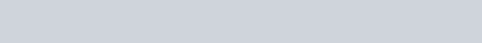The fixed assets are considered at their book value and the proprietor’s funds consist of the same items as internal equities in the case of debt equity ratio.

Interest Coverage Ratio :This ratio deals only with servicing of return on loan as interest. This ratio depicts the relationship between amount of profit utilise for paying interest and amount of interest payable. A high Interest Coverage Ratio implies that the company can easily meet all its interest obligations out of its profit.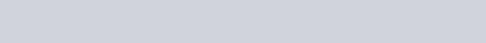Question 4. What are important profitability ratios? How are they worked out? ‘
Answer Profitability Ratios Profitability ratios measure the results of business operations or overall performance and effectiveness of the firm. Some of the most Important and popular profitability ratios are as under
Gross Profit Ratio: Gross Profit Ratio (GP ratio) is the ratio of gross profit to net sales expressed as a percentage. It expresses the relationship between gross profit and sales. The basic components for the calculation of gross profit ratio are gross profit and net sales. Net sales mean sales minus sales returns.

Gross profit would be the difference between net sales and cost of goods sold. Cost of goods sold in the case of a trading concern would be equal to opening stock plus purchase, minus closing stock plus all direct expenses relating to purchases. In the case of manufacturing concern, it would be equal to the sum of the cost of raw materials, wages, direct expenses and all manufacturing expenses. In other words, generally the expenses charged to profit and loss account or operating expenses are excluded from the calculation of cost of goods sold.
Following formula is used to calculate gross profit ratiosNet Profit Ratio :Net Profit Ratio is the ratio of net profit to net sales. It is expressed as percentage. The two basic components of the net profit ratio are the net profit and sales. The net profits are obtained after deducting income-tax and, generally, non-operating expenses and incomes are excluded from the net profits for calculating this ratio. Thus, incomes such as interest on investments outside the business, profit on sales of fixed assets and losses on sales of fixed assets, etc are excluded.Operating Profit Ratio :Operating Profit Ratio is the ratio of operating profit to net sales. There are many non operating expenses and incomes included in the profit and loss account which has nothing to do with the operations of the business such as loss by fire, loss by theft etc. On the other had in credit side of the P&L account, there are so many incomes
which can be considered as operating incomes such as dividend, bank interest, rent etc. In this way net profit ratio will not tell the truth about the profit of the organisation. Hence operating profit ratio will be helpful in that case. The formula for calculating operating ratio is as followsOperating Ratio :Operating ratio is the ratio of cost of goods sold plus operating expenses to net sales. It is generally expressed in percentage, Operating ratio measures the cost of operations per dollar of sales. This is closely related to the ratio of operating profit to net sales. The two basic components for the calculation of operating ratio are operating cost (cost of goods sold plus operating expenses) and net sales. Operating expenses normally include (a) administrative and office expenses and (b) selling and distribution expenses. The formula for calculating the operating ratio is as followsQuestion 5. Financial ratio analysis are conducted by four groups of analysts : managers, equity investors, long term creditors and short term creditors. What is the primary emphasis of each of these groups in evaluating ratios?
Answer This is very much true that the financial ratio analysis is conducted by four groups of analysts : managers, equity investors, long term creditors and short term creditors. The primary emphasis of each of these groups in evaluating these ratios are as follows
(i) Management: Management calculate ratios for taking various managerial decisions. Management is always interested in future growth of the organisation. In this regard management design various policy measures and draft future plans. Management wish to know how effectively the resources are being utilised Consequently, they are interested in Activity Ratios and Profitability Ratios like Net Profit Ratio, Debtors Turnover Ratio, Fixed Assets Turnover Ratios, etc.
(ii) Equity Investors: The prime concern of investors before investing in shares is to ensure the security of their principle and return on investment. It is a well known fact that the security of the funds is directly related to the profitability and operational efficiency of the business. In this way they are interested in knowing Earnings per Share, Return on Investment and Return on Equity.
(iii) Long Term Creditors: Long term creditors are those creditors who provide funds for more than one year, so they are interested in long term solvency of the firm and in assessing the ability of the firm to pay interest on time. In this way they are interested in calculating Long term Solvency Ratios like, Debt-Equity Ratio, Proprietory Ratio, Total Assets to Debt Ratio, Interest Coverage Ratio, etc.
(iv) Short Term Creditors: Short term creditors are those creditors who provide financial assistance through short term credit (Generally less than one year). That’s why short term creditors are interested in timely payment of their debts in short run. In this way, they are always interested in Liquidity Ratios like, Current Ratio, Quick Ratios etc. These ratios reveal the current financial position of the business. It is always observed that short term obligations are paid through current assest.

Question 6. The current ratio provides a better measure of overall liquidity only when a firm’s inventory cannot easily be converted into cash. If inventory is liquid, the quick ratio is a preferred measure of overall liquidity. Explain.
Answer The above mentioned statement is true. There are two different ways to measure the liquidity of a firm first through current ratio of the firm and second through quick ratio of the firm. The second one is considered the more refine form of measuring the liquidity of the firm.
The current ratio ‘explains the relationship between current assets and current liabilities. If current assets are quite capable to pay the current liability the liquidity of the concerned firm will be considered good. But here generally one question arises there are certain assets which cannot be converted into cash quickly such as stock and prepaid expenses.
As far as the matter of prepaid expenses is concerned it’s ok but what about the stock if we measure the liquidity on the basis of conversion of current assets in cash there are many firms where conversion of stock is not possible into cash frequently say e.g., heavy machinery manufacturing companies, locomotive companies, etc. This is because, the heavy stocks like machinery, heavy tools etc. cannot be easily sold off. In this case it is always advisable to follow the current ratio for measuring the liquidity of a firm.

But on the other hand, in case of those firms where the stock can be easily realised or sold off consideration of stock should be avoided and to measure the liquidity of that firm Quick ratio should be calculated, e.g., the inventories of a service sector company are very liquid as there are no stocks kept for sale, so in that case liquid ratio must be followed for measuring the liquidity of the firm.
We can understand from the above mentioned statement in the light of another example where stock contribute the major portion in current assets in that case to find out the liquidity of that firm stock cannot be avoided to measure the liquidity of the firm. On the other hand where stock contributes a reasonably less amount it can be avoided and liquidity of that firm can be measured with the help of quick ratio. On the other hand where there is a lot of fluctuation in the price of stock it is always advisable to compute quick ratio and avoid the stock figure because it will reduce the authenticity of liquidity measure.

### NUMERICAL QUESTIONS

Question 1. Following is the Balance Sheet of Rohit and Company as on March 31, 2006.Calculate Current Ratio.Question 2. Following is the Balance Sheet of Title Machine Limited as on March 31, 2006.Calculate Current Ratio and Liquid Ratio.Question 3. Current Ratio is 3:5 Working Capital is Rs. 9,00,000. Calculate the amount of Current Assets and Current Liabilities.Note :According to the ratio, current asset is less than current liability hence working capital should be negative. To match the figures and answer of the question current ratio is taken as 3.5 : 1 and working capital ? 90,000.Question 4. Shine Limited has a current ratio 4.5:1 and quick ratio 3:1; if the stock is 36,000, calculate current liabilities and current assets.Question 5. Current liabilities of a company are Rs. 75,000. If Current ratio is 4 : 1 and liquid ratio is 1:1, calculate value of current assets, liquid assets and stock.Question 6. Handa Limited has stock of Rs. 20,000. Total liquid assets are Rs. 1,00,000 and quick ratio is 2:1 Calculate current ratio.Question 7. Calculate debt equity ratio from the following informationQuestion 8. Calculate Current Ratio if Stock is ? 6,00,000; Liquid Assets Rs. 24,00,000; Quick Ratio 2:1.Question 9. Compute Stock Turnover Ratio from the following informationQuestion 10. Calculate following ratios from the following information
(i) Current ratio (ii) Acid test ratio
(iii) Operating Ratio (iv) Gross Profit RatioNote (i) Acid test ratio, quick ratio and liquid ratio are one and the same.
(ii) Students mostly get confused in operating ratio and operating profit ratio, so be careful while doing these ratios.

Question 11. From the following information calculate
(i) Gross Profit Ratio (ii) Inventory Turnover Ratio (iii) Current Ratio (iv) Liquid Ratio
(v) Net Profit Ratio (vi) Working Capital RatioNote :In this question stock is given separately from current assets, hence* it is added to make total current assets.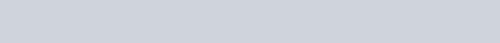Note :In this question current assetts should be considered as other current asset and stock is separate, in other words, other current assets means liquid assets. Working capital ratio and working capital turnover ratio means same.

Question 12. Compute Gross Profit Ratio, Working Capitat Turnover Ratio, Dept Equity Ratio and Proprietory Ratio from the fottowing informationQuestion 13. Calculate Stock Turnover Ratio if Opening Stock is Rs. 76,250, Closing Stock is 98,500, Sales is Rs. 5,20,000, Sales Return is Rs.20,000, Purchase is Rs. 3,22,250.Question 14. Calculate Stock Turnover Ratio from the data given belowQuestion 15. A trading firm’s average stock is ? 20,000 (cost). If the stock turnover ratio is 8 times and the firm setts goods at a profit of 20% on sales, ascertain the profit of the firm.Question 16. You are able to collect the following information about a company for two yearsCalculate Stock Turnover Ratio and Debtor Turnover Ratio if in the year 2004 stock in trade increased by Rs. 2,00,000.Question 17. The following Balance Sheet and other information, calculate following ratios
(i) Debt Equity Ratio (ii) Working Capital Turnover Ratio
(iii) Debtors Turnover RatioQuestion 18. The following is the summarised Profit and Loss account and the Balance Sheet of Nigam Limited for the year ended March 31, 2007Calculate
(i) Quick Ratio
(ii) Stock Trunover Ratio
(iii) Return on Investment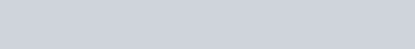Question 19. From the following,
Calculate
(a) Debt Equity Ratio (b) Total Assets to Debt Ratio (c) Propietory Ratio.Question 20. Cost of Goods Sold is 1 1,50,000 Operating expenses are Rs. 60,000. Sales is Rs. 2,60,000 and Sales Return is Rs. 10,000. Calculate Operating Ratio.Question 21. The following is the summerised transactions and Profit and Loss Account for the year ending March 31, 2007 and the Balance Sheet as on that date.Calculate (i) Gross Profit Ratio (ii) Current Ratio (iii) Acid Test Ratio
(iv) Stock Turnover Ratio (v) Fixed Assets Turnover Ratio.Question 22. From the fotlowing information calcutate Gross Profit Ratio, Stock Turnover Ratio and Debtors Turnover Ratio.